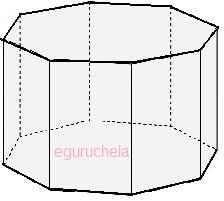#### Octogonal prism Area, Volume & Surface Area Calculation

Calculates the octagonal-prism area, octagonal-prism volume and octagonal-prism surface area for given side length(a), height(l) and distance(d) using the following formulas:
Area (A)               = 2 X side-length(a) X distance(d)
Volume (V)          = Area(A) X height(l)
Surface Area (SA) = (2 X A) + (8 X a X l)Please enter Side Length(a) = Please enter Height (l) = Please enter Distance (d) = Area (A) = Volume (V) = Surface Area (SA) =
The octagonal prism is solid (3-dimensional) object having total 10 faces, 24 edges and 16 vertices. The octagonal prism has rectangle side shape and octagonal shape for top & base.